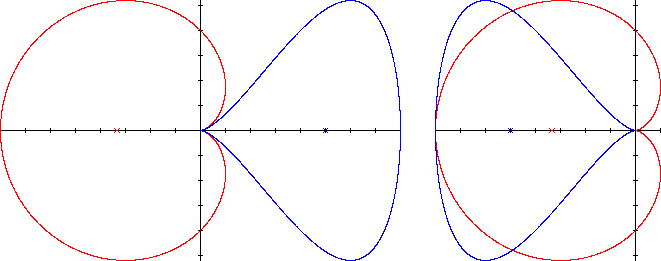Cardioid
CARDIOID

Balmoral Software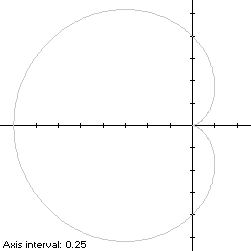A cardioid having its cusp on the right is a heart-shaped x-symmetric closed curve S with polar equation
r(t) = 1 - cos(t), 0 ≤ t < 2π
The curve is traced out in a counterclockwise direction, starting from the cusp at the origin. Its minimum abscissa occurs at the point (-2,0) when t = π. Its maximum abscissas occur at the points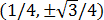when t = ±π/3, which determine that the cardioid is non-convex by the multiple local extrema test. The maximum ordinate of the cardioid occurs at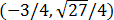when t = 2π/3, so the width x height of its bounding rectangle is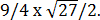### Metrics

The perimeter of the cardioid is 8 and its area is 3π/2. Its centroid is the point (-5/6,0). The bounding rectangle for inconics is delimited by the cusp at the origin.

### Convex Hull

The convex hull is created by connecting the maximum abscissa points with a vertical line segment of length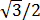at x = 1/4. We have
r'(t) = sin(t),
so by (L2), the perimeter of the convex hull is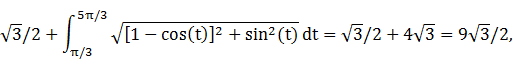which is about 3% shorter than that of the cardioid.

The line segment of the convex hull creates an isosceles triangle with the origin, having area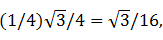as shown in blue in the left diagram below. The centroid abscissa of this triangle is the average of its vertex abscissas, or 1/6. By (A2), the remainder of the convex hull has area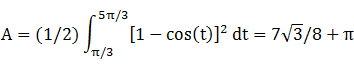By (C2), the associated centroid abscissa is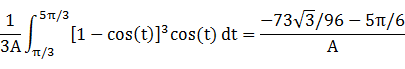The convex hull component metrics can be summarized as follows:
 Area Centroid abscissa Product Region Triangle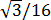1/6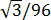Remainder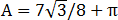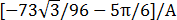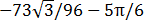Total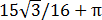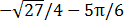The area of the convex hull is 4.765390, about 1% larger than that of the cardioid. The centroid abscissa of the convex hull is the weighted average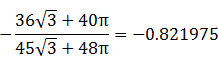### Incircle

A candidate incircle is located on the x-axis between the minimum abscissa -2 and the cusp at the origin, and so has radius R = 1 and center abscissa c = -1. For verification, we have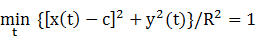### Inellipse

Using z = 0 in Lemma E,
d/dt [x(t) - z]y(t) = d/dt [1 - cos(t)]2sin(2t)/2
has a zero at t* = 4π/5. The corresponding coordinates are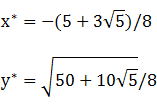We then have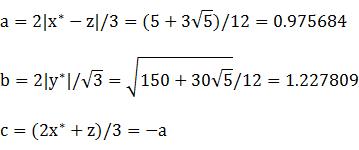For verification,### Circumellipse

Using z = -2 in Lemma E,
d/dt [x(t) - z]y(t) = d/dt sin3(t)[2 - cos(t)]
has a zero at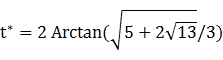The corresponding coordinates are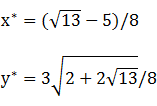We then have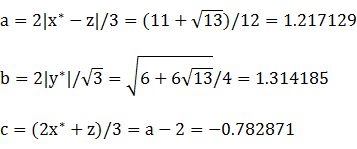For verification,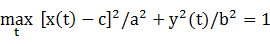### Circumcircle

The radius of a circle centered on the x-axis and circumscribing S is at least its maximum ordinate, so a candidate for the circumcircle has radius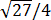and center abscissa c = -3/4. For verification,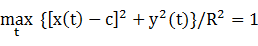### Summary Table

 Perimeter Area Centroid Figure Parameters Incircle R = 1 6.283185 3.141593 (-1,0) Inellipse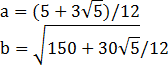6.945153 3.763482 (-0.975684,0) Cardioid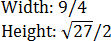8 4.712389 (-0.833333,0) Convex hull 7.794229 4.765390 (-0.821975,0) Circumellipse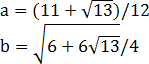7.955280 5.025080 (-0.782871,0) Circumcircle R =8.162097 5.301438 (-0.75,0)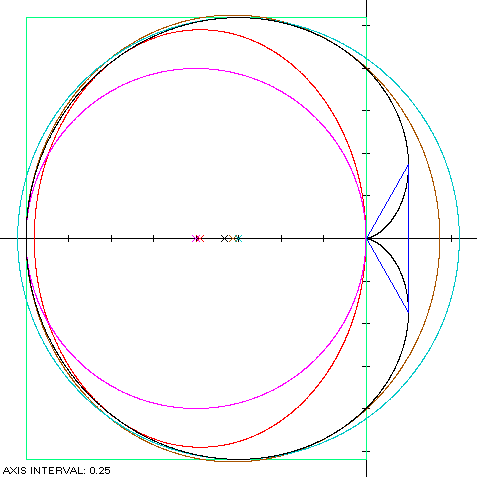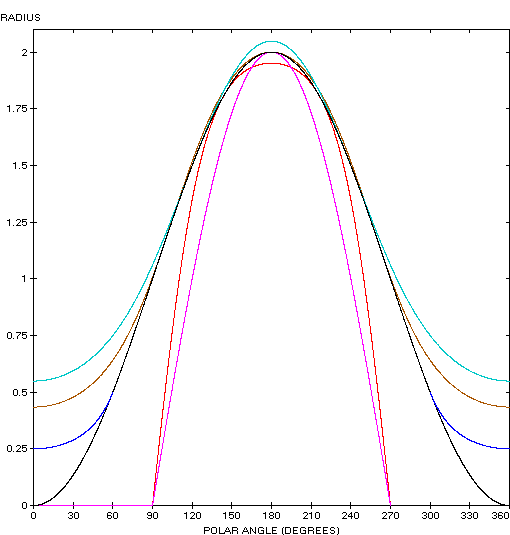The cardioid (red) is a member of a group of similarly-shaped figures described on these pages, including (inside to outside) the rotated lima bean curve, the cochleoid and the Cayley sextic: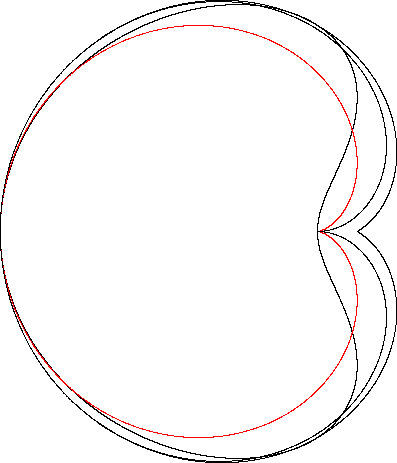The cardioid adjoins and is the same height as the piriform curve: